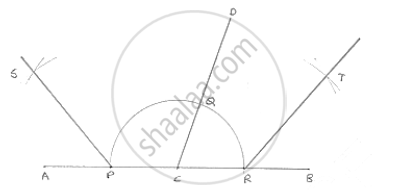# Draw a Linear Pair of Angles. Bisect Each of the Two Angles. Verify that the Two Bisecting Rays Are Perpendicular to Each Other. - Mathematics

Draw a linear pair of angles. Bisect each of the two angles. Verify that the two bisecting rays are perpendicular to each other.

#### SolutionSteps of construction:
1. Draw two angle DCA and DCB forming Linear pair
2. With center C and any radius, draw an arc which intersects AC at P, CD at Q and CB at R.
3. With center P and Q and any radius draw two arcs which interest each other at S
4. Join SC
5. With center Q and R any radius draw two arcs, which intersect each other at T.
6. Join TC
∠SCT = 90°                   [By using protractor]

Concept: Basic Constructions
Is there an error in this question or solution?

#### APPEARS IN

RD Sharma Mathematics for Class 9
Chapter 16 Constructions
Exercise 16.2 | Q 5 | Page 9

Share